# 从环境搭建到回归神经网络案例，带你掌握Keras

## 一.为什么要使用Keras

Keras是一个由Python编写的开源人工神经网络库，可以作为Tensorflow、Microsoft-CNTK和Theano的高阶应用程序接口，进行深度学习模型的设计、调试、评估、应用和可视化 。其主要开发者是谷歌工程师François Chollet。

Keras在代码结构上由面向对象方法编写，完全模块化并具有可扩展性，其运行机制和说明文档有将用户体验和使用难度纳入考虑，并试图简化复杂算法的实现难度 。Keras支持现代人工智能领域的主流算法，包括前馈结构和递归结构的神经网络，也可以通过封装参与构建统计学习模型。在硬件和开发环境方面，Keras支持多操作系统下的多GPU并行计算，可以根据后台设置转化为Tensorflow、Microsoft-CNTK等系统下的组件。

Keras作为神经网络的高级包，能够快速搭建神经网络，它的兼容性非常广，兼容了TensorFlow和Theano。

## 二.安装Keras和兼容Backend

### 1.如何安装Keras

• Numpy
• Scipy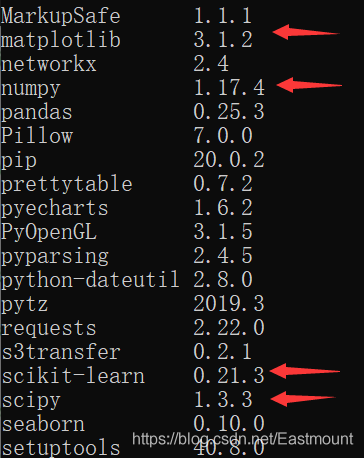```activate tensorflow
pip3 install keras
pip install keras```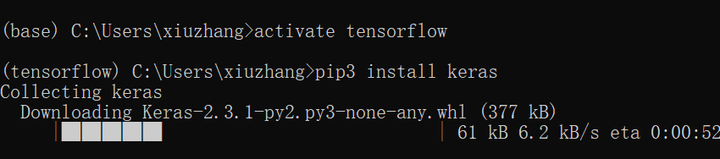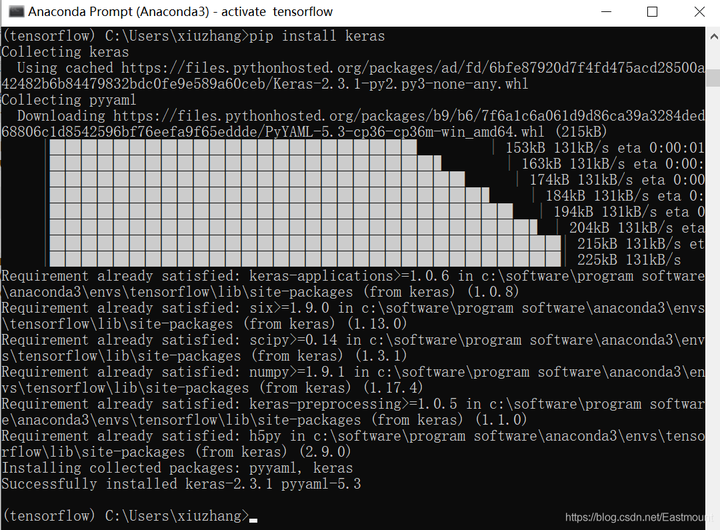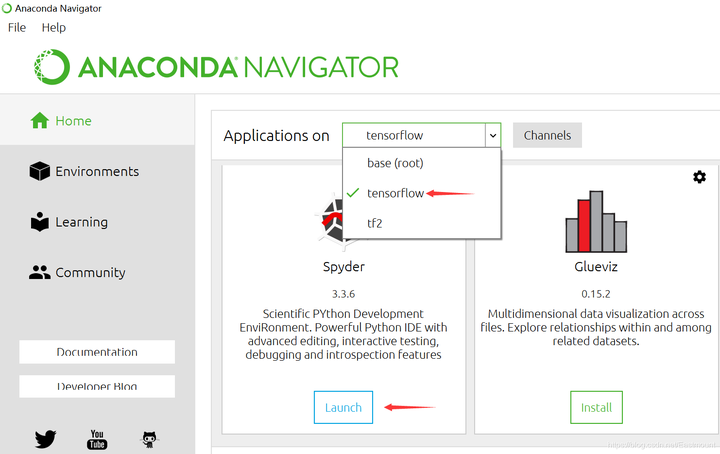```# -*- coding: utf-8 -*-
"""
Created on Fri Feb 14 16:43:21 2020
@author: Eastmount CSDN
"""
import numpy as np
from keras.preprocessing.sequence import TimeseriesGenerator

# 时间序列
y = np.array(range(5))
tg = TimeseriesGenerator(y, y, length=3, sampling_rate=1)
for i in zip(*tg):
print(*i)```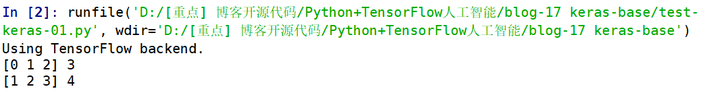### 2.兼容Backend

Backend是指Keras基于某个框架来做运算，包括基于TensorFlow或Theano，上面的那段代码就是使用TensorFlow来运算的。后面要讲解的神经网络也是基于TensorFlow或Theano来搭建的。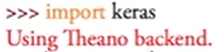• 第一种方法，找到“keras/keras.json”这个文件，然后打开它。所有的backend信息就存储在这里，每次导入Keras包就会检测这个“keras.json”文件的backend。接着我们尝试修改。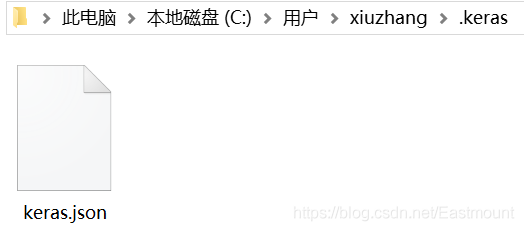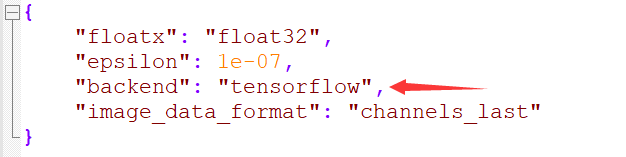• 第二种方法是在命令行中输入下面这句命令，每次运行脚本时它会直接帮你修改成临时的TensorFlow。
```import os
os.environ['KERAS_BACKEND']='tensorflow'
import keras```

## 三.白话神经网络### 首先，什么是神经网络（Neural Networks）？### 神经网络是如何工作的呢？

• 输入层：直接接收信息的神经层，比如接收一张猫的图片
• 输出层：信息在神经元中传递中转和分析权衡，形成输出结果，通过该层输出的结果可以看出计算机对事物的认知
• 隐藏层：在输入和输出层之间的众多神经元连接组成的各个层面，可以有多层，负责对传入信息的加工处理，经过多层加工才能衍生出对认知的理解### 神经网络举例说明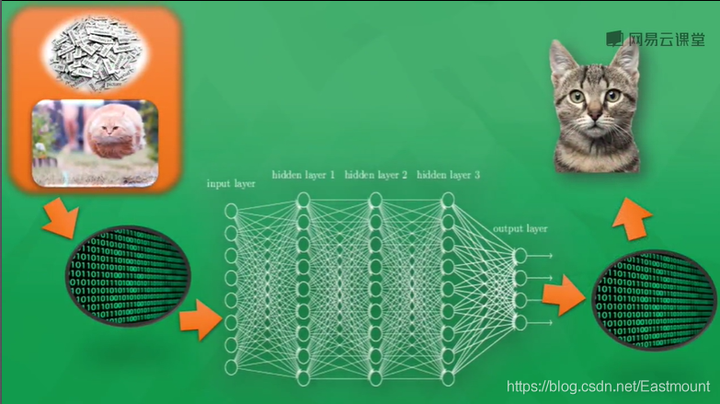### 计算机是怎么训练的呢？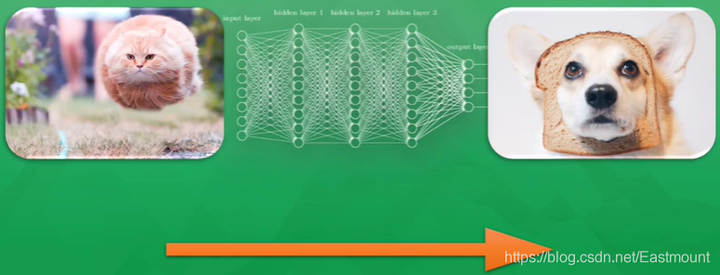### 那么计算机是如何学习经验的呢？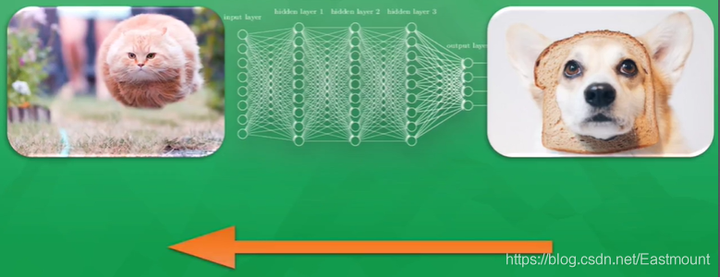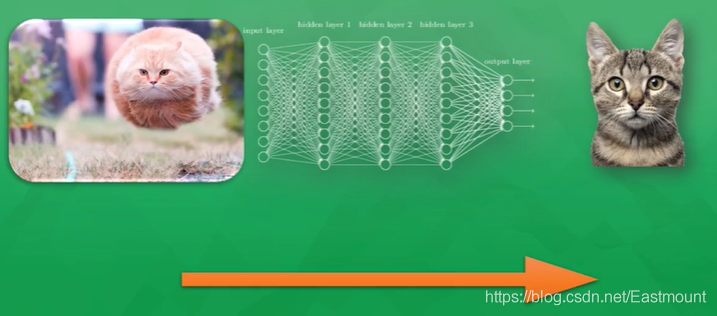### 激励函数是什么东东？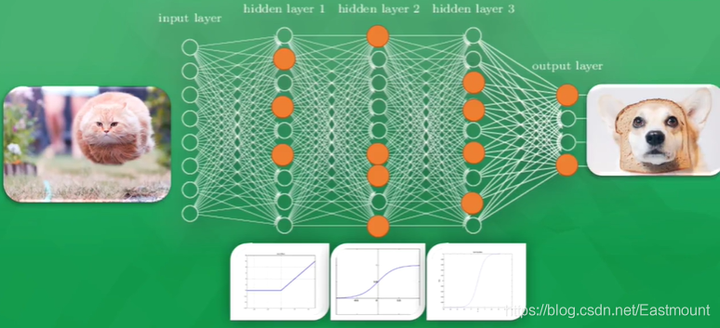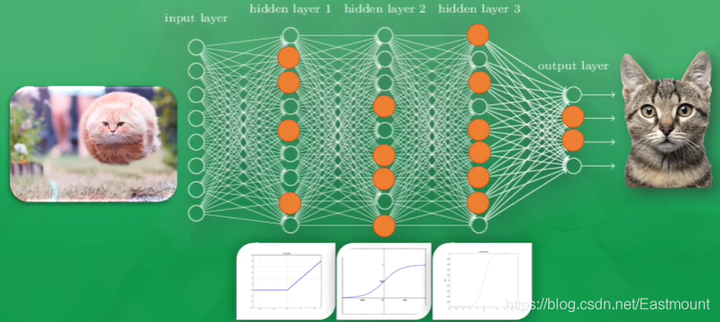## 四.Keras搭建回归神经网络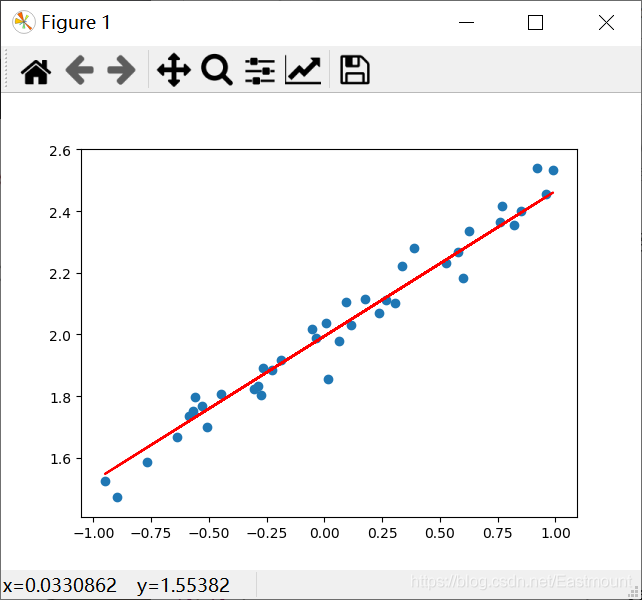### 1.导入扩展包

Sequential（序贯模型）表示按顺序建立模型，它是最简单的线性、从头到尾的结构顺序，不分叉，是多个网络层的线性堆叠。Dense是layers中的属性，表示全连接层。Keras还可以实现各种层，包括core核心层、Convolution卷积层、Pooling池化层等非常丰富有趣的网络结构。

```import numpy as np
from keras.models import Sequential
from keras.layers import Dense
import matplotlib.pyplot as plt```

### 2.创建散点图数据

```import numpy as np
from keras.models import Sequential
from keras.layers import Dense
import matplotlib.pyplot as plt

#---------------------------创建散点数据---------------------------
# 输入
X = np.linspace(-1, 1, 200)
# 随机化数据
np.random.shuffle(X)
# 输出
y = 0.5*X + 2 + np.random.normal(0, 0.05, (200,)) #噪声平均值0 方差0.05
# 绘制散点图
plt.scatter(X, y)
plt.show()

# 数据集划分(训练集-测试集)
X_train, y_train = X[:160], y[:160]  # 前160个散点
X_test, y_test = X[160:], y[160:]    # 后40个散点```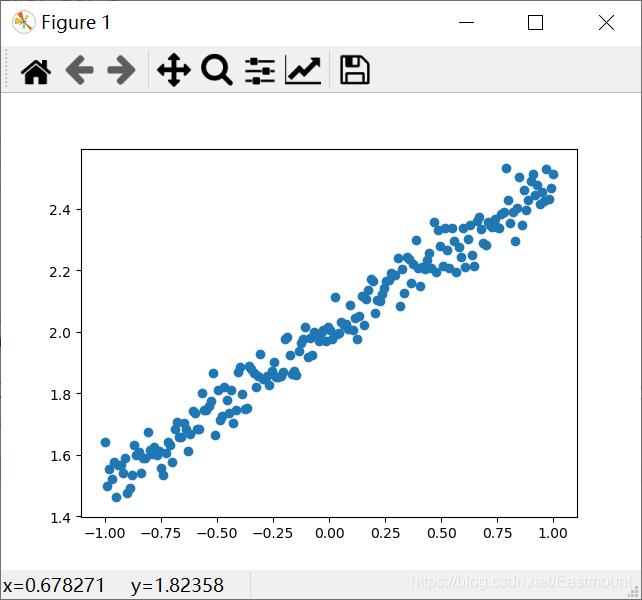### 3.添加神经网络层

• 创建Sequential模型。
• 搭建模型并选择损失函数(loss function)和优化方法(optimizing method)。
```#----------------------------添加神经层------------------------------
# 创建模型
model = Sequential()

# 增加全连接层 输出个数和输入个数(均为1个)

# 搭建模型 选择损失函数(loss function)和优化方法(optimizing method)
# mse表示二次方误差 sgd表示乱序梯度下降优化器
model.compile(loss='mse', optimizer='sgd')```

PS：是不是感觉Keras代码比TensorFlow和Theano都简洁很多，但还是建议大家先学习前者，再深入Keras。

### 4.训练并输出误差

```print("训练")
# 学习300次
for step in range(301):
# 分批训练数据 返回值为误差
cost = model.train_on_batch(X_train, y_train)
# 每隔100步输出误差
if step % 100 == 0:
print('train cost:', cost)```

### 5.测试神经网络并输出误差\权重和偏置

```print("测试")
# 运行模型测试 一次传入40个测试散点
cost = model.evaluate(X_test, y_test, batch_size=40)
# 输出误差
print("test cost:", cost)
# 获取权重和误差 layers表示第一个神经层(即Dense)
W, b = model.layers.get_weights()
# 输出权重和偏置
print("weights:", W)
print("biases:", b)```

### 6.绘制预测图形

```y_pred = model.predict(X_test)
plt.scatter(X_test, y_test)
plt.plot(X_test, y_pred)
plt.show()``````训练
train cost: 4.002261
train cost: 0.07719966
train cost: 0.005076804
train cost: 0.0030148015

40/40 [==============================] - 0s 1ms/step
test cost: 0.0028453178238123655
weights: [[0.47052705]]
biases: [1.9944116]```

```# -*- coding: utf-8 -*-
"""
Created on Fri Feb 14 16:43:21 2020
@author: Eastmount CSDN YXZ
O(∩_∩)O Wuhan Fighting!!!
"""
import numpy as np
from keras.models import Sequential
from keras.layers import Dense
import matplotlib.pyplot as plt

#---------------------------创建散点数据---------------------------
# 输入
X = np.linspace(-1, 1, 200)
# 随机化数据
np.random.shuffle(X)
# 输出
y = 0.5*X + 2 + np.random.normal(0, 0.05, (200,)) #噪声平均值0 方差0.05
# 绘制散点图
# plt.scatter(X, y)
# plt.show()

# 数据集划分(训练集-测试集)
X_train, y_train = X[:160], y[:160]  # 前160个散点
X_test, y_test = X[160:], y[160:]    # 后40个散点

#----------------------------添加神经层------------------------------
# 创建模型
model = Sequential()

# 增加全连接层 输出个数和输入个数(均为1个)

# 搭建模型 选择损失函数(loss function)和优化方法(optimizing method)
# mse表示二次方误差 sgd表示乱序梯度下降优化器
model.compile(loss='mse', optimizer='sgd')

#--------------------------------Traning----------------------------
print("训练")
# 学习300次
for step in range(301):
# 分批训练数据 返回值为误差
cost = model.train_on_batch(X_train, y_train)
# 每隔100步输出误差
if step % 100 == 0:
print('train cost:', cost)

#--------------------------------Test-------------------------------
print("测试")
# 运行模型测试 一次传入40个测试散点
cost = model.evaluate(X_test, y_test, batch_size=40)
# 输出误差
print("test cost:", cost)
# 获取权重和误差 layers表示第一个神经层(即Dense)
W, b = model.layers.get_weights()
# 输出权重和偏置
print("weights:", W)
print("biases:", b)

#------------------------------绘制预测图形-----------------------------
y_pred = model.predict(X_test)
plt.scatter(X_test, y_test)
plt.plot(X_test, y_pred, "red")
plt.show()```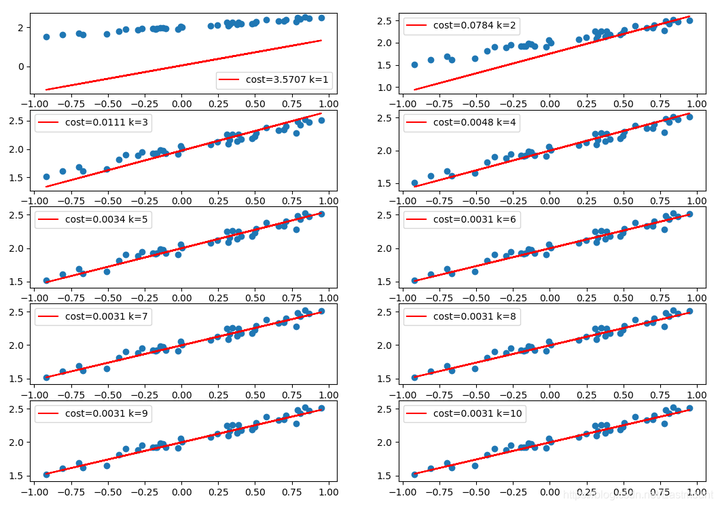```# -*- coding: utf-8 -*-
"""
Created on Fri Feb 14 16:43:21 2020
@author: Eastmount CSDN YXZ
"""
import numpy as np
from keras.models import Sequential
from keras.layers import Dense
import matplotlib.pyplot as plt

#---------------------------创建散点数据---------------------------
# 输入
X = np.linspace(-1, 1, 200)
# 随机化数据
np.random.shuffle(X)
# 输出
y = 0.5*X + 2 + np.random.normal(0, 0.05, (200,)) #噪声平均值0 方差0.05
# 绘制散点图
# plt.scatter(X, y)
# plt.show()

# 数据集划分(训练集-测试集)
X_train, y_train = X[:160], y[:160]  # 前160个散点
X_test, y_test = X[160:], y[160:]    # 后40个散点

#----------------------------添加神经层------------------------------
# 创建模型
model = Sequential()

# 增加全连接层 输出个数和输入个数(均为1个)

# 搭建模型 选择损失函数(loss function)和优化方法(optimizing method)
# mse表示二次方误差 sgd表示乱序梯度下降优化器
model.compile(loss='mse', optimizer='sgd')

#--------------------------------Traning----------------------------
print("训练")
k = 0
# 学习1000次
for step in range(1000):
# 分批训练数据 返回值为误差
cost = model.train_on_batch(X_train, y_train)
# 每隔100步输出误差
if step % 100 == 0:
print('train cost:', cost)

#-----------------------------------------------------------
# 运行模型测试 一次传入40个测试散点
cost = model.evaluate(X_test, y_test, batch_size=40)
# 输出误差
print("test cost:", cost)
# 获取权重和误差 layers表示第一个神经层(即Dense)
W, b = model.layers.get_weights()
# 输出权重和偏置
print("weights:", W)
print("biases:", b)

#-----------------------------------------------------------
# 可视化绘图
k = k + 1
plt.subplot(5, 2, k)
y_pred = model.predict(X_test)
plt.scatter(X_test, y_test)
plt.plot(X_test, y_pred, "red", label='cost=%.4f k=%d' %(cost,k))
plt.legend()

plt.show()```